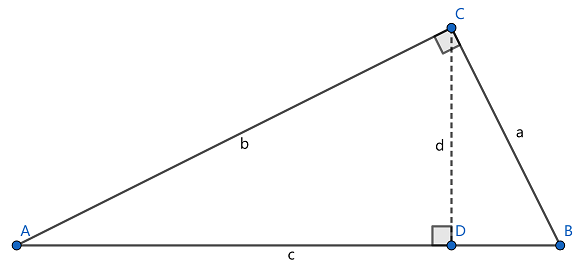安卓手机扫描二维码安装AppA. ﻿$\dfrac{1}{a^2}+\dfrac{1}{d^2}=\dfrac{1}{b^2}$﻿

B. ﻿$\dfrac{1}{a^2}+\dfrac{1}{b^2}=\dfrac{1}{d^2}$﻿

C. ﻿$\dfrac{1}{b^2}+\dfrac{1}{c^2}=\dfrac{1}{a^2}$﻿

D. ﻿$\dfrac{1}{b^2}+\dfrac{1}{d^2}=\dfrac{1}{a^2}$﻿苹果手机扫描二维码安装App